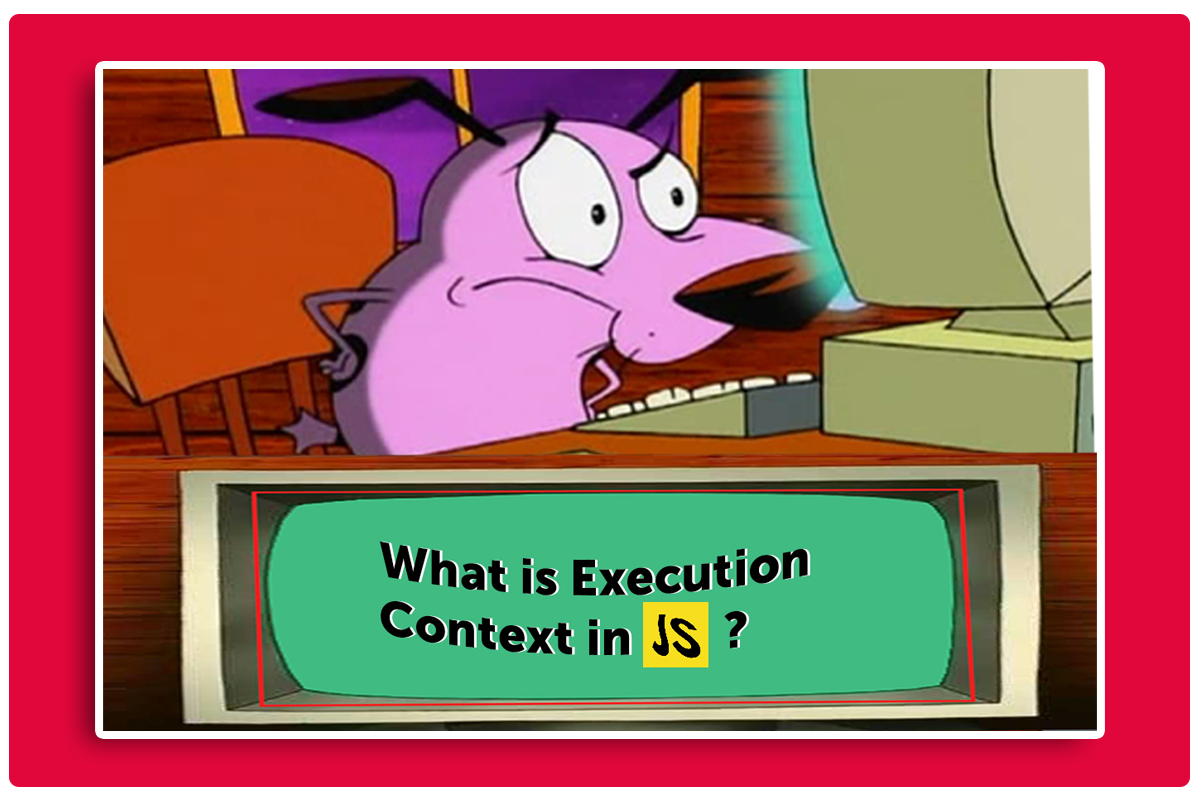Engineering
May 19, 2022

# Execution Context: How JavaScript works!Everything in JS happens inside the execution context.

Now, what exactly is execution context?

It is an environment that runs and stores your javascript code. It is created when your code is executed. This environment has access to “this”, variables objects, and functions of your javascript code. Whenever any code runs in javascript, it happens inside an execution context.

It is the first thing that is created when we write javascript code.

Types of execution context:

• Global Execution context (GEC)
• Function Execution context (FEC)

So consider this figure as the execution context which is divided into two parts.

The first part is known as the memory component or variable environment, this is the place where all of your functions and variables are stored in key-value pairs. The second part of the execution context is known as the thread of execution or you can consider it as your code component, this is the place where your javascript code is executed [one line at a time].

It is important to know here that javascript is a single-threaded and synchronous language. This basically means that javascript can execute one line of code at a time following a specific order.

Let’s dive into this code example to understand how this execution context works.

Here we have two variables l and w (short for length and width) on line 1 and 2 and then we have this function calculate which takes length, and width as its parameters that calculates the area of a rectangle and returns it. And on line 9 we have the variable rectangle1 which is invoking the calculate function providing the arguments (l & w).

Now let us understand how this javascript code runs behind the scenes.

As we know this execution context is created in two phases:

• Memory creation phase
• Code execution phase

### Memory creation phase:

Now the first case which is the memory creation phase, javascript here will allocate memory to all the variables and functions.

On line 1 and 2 we have two variables l & w as soon as javascript encounters them, memory is allocated to both of these in the memory phase and both of them are given a value of undefined.

On line 4 javascript sees this calculate function so it allocates some memory to calculate and stores the whole code of the calculate function as its value.

On line 9 we have a variable rectangle1, javascript will allocate memory to it and store undefined as its value.

So in the first phase [memory creation phase] javascript allocates memory. Notice that it stores the whole code in case of a function and stores undefined for the variable as their value. We will discuss why these variables have undefined as their value in my upcoming blogs, for now, let's keep things plain and simple.

### Code execution phase:

Now let’s talk about the second phase the code execution phase. So once again javascript goes through this program line by line and this is the execution phase where all logic and calculations are done.

On line 1 the identifier l will have its value as 2 now and on line 2 the identifier w will have its value as 3.

Javascript then skips lines [4 -7] as we have nothing to do and execute there.

Now, this is where it gets interesting on line 9 we are calling or invoking a function. now we have to understand that function itself are mini-programs and when a function is invoked a completely new execution context is created which is known as function execution context (FEC).

On line number 9, a separate functional execution context will be created which again has two phases the memory and code execution phase. Now we have to execute the piece of code inside the function.

So, let's see how the memory is allocated here, in the first phase we assign memory to variables and functions and this piece of code does not have functions so we don’t have to worry about that.

### FEC: Memory Phase

So, starting from the parameters we will assign memory to length & width variables and on line 5 we will also allocate memory to the area as well. All these variables will have undefined as their value as discussed earlier.

### FEC: code execution phase

We will be executing each line from the point of execution i.e where the function is invoked.

where the l and w are passed as arguments to the parameters length&width having values 2 and 3. So the value of length and width will be changed to 2 and 3 instead of undefined.

On line 5, javascript will execute/calculate this area which is length*width and store the computed value to the area in the memory block. So now the area will be 6.

On line number 6 javascript encounters the return keyword where we are returning the area which essentially tells javascript that we are done with this function ****and now we have to return the whole control back to the execution context where the function was invoked. Which in our case is the global execution context (GEC).

On line number 6 as soon as we encounter the return keyword with area, javascript will look for that area variable inside the local memory and return its value i.e 6 back to the execution context where the function was invoked.

So giving the control back to the GEC we will have the value 6 stored inside rectangle1 variable instead of undefined on line number 9.

One important thing to remember: is that when the whole function is executed the function execution context created for that function instance will be deleted

Phew! So now that line number 9 is executed! javascript is done with the execution and the program is finished which means the whole global execution context is now deleted.